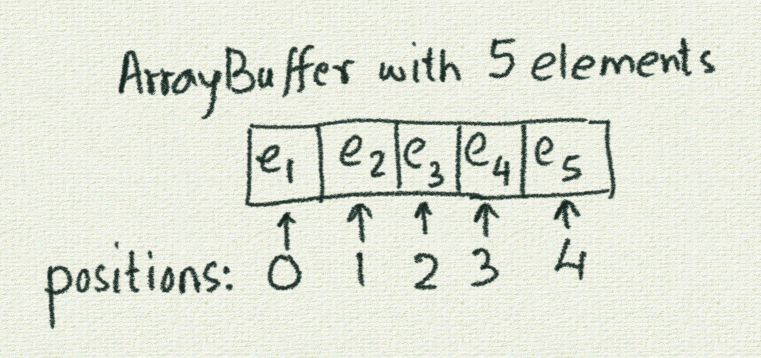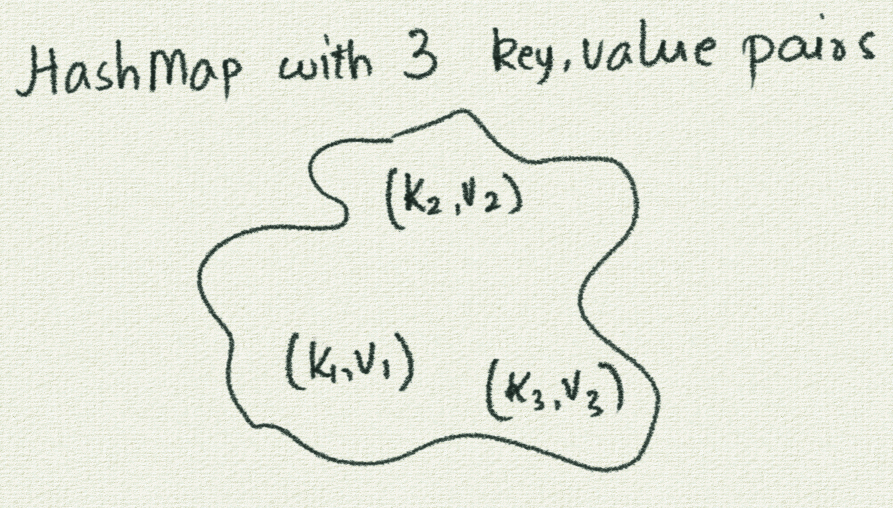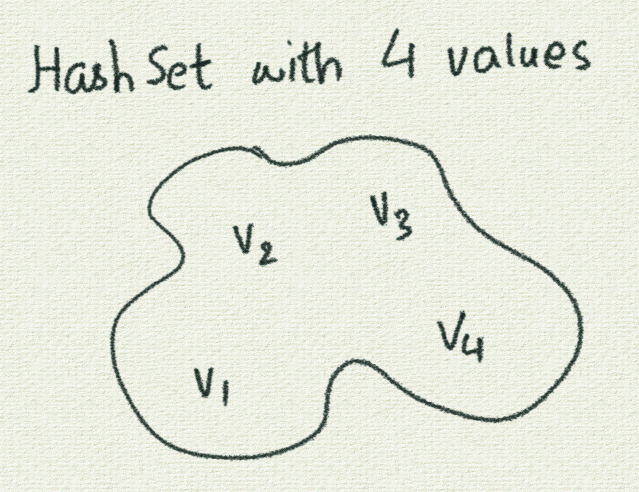## Quickref - ArrayBuffer, HashMap, and HashSet for gaming

1 ArrayBuffer

An ArrayBuffer is a sequence of elements. Every element can be located based on its position or index within the ArrayBuffer.

Positions (or indices) start from `0` and go upto `length - 1`.

Here is a pictorial representation of an ArrayBuffer:The following are the primary operations that you can do with an ArrayBuffer:

1.1 Creation

• `ArrayBuffer.empty[type]` - creates an empty ArrayBuffer which will hold elements of the given type.

Example:

``````ArrayBuffer.empty[Int]
ArrayBuffer.empty[Picture]
``````
• `ArrayBuffer(e1, e2, e3)` - creates an ArrayBuffer with the given elements

Example:

``````ArrayBuffer(4, 9, 7)
ArrayBuffer(pic1, pic2, pic3, pic4)
``````

1.2 Addition of elements at the end

• `ab.append(element)` - adds `element` to the end of an ArrayBuffer called `ab`.

Assume that you have created an ArrayBuffer of Ints called `ab1` and and ArrayBuffer of Pictures called `ab2`, and that you have a Picture called `pic5`.

Example:

``````ab1.append(7)
ab2.append(pic5)
``````

1.3 Iteration over all the elements

Iterating over the elements means going over all the elements (to do something with each)

• `repeatFor(ab) { e => /* do whatever with e /* }` - iterators over all the elements of an ArrayBuffer called `ab`. While iterating, the current element is called `e` in the given example. Your code can do whatever it wants with `e`.

Assume that you have created an ArrayBuffer of Ints called `ab1` and an ArrayBuffer of Pictures called `ab2`.

Example:

``````repeatFor(ab1) { n =>
println(n)
}

repeatFor(ab2) { p =>
draw(p)
}
``````

2 HashMap

A HashMap is a collection of key-value pairs. You can add key-value pairs to a HashMap, and quickly look up the values for given keys.

Here is a pictorial representation of a HashMap:The following is a description of the primary operations that you can do with a HashMap:

2.1 Creation

• `HashMap.empty[type1, type2]` - creates an empty HashMap which will hold keys of `type1` and values of `type2`.

Example:

``````HashMap.empty[String, Int]
HashMap.empty[Picture, Vector2D]
``````

2.2 Addition of key, value pairs

• `hm.(key) = value` - adds the pair `(key, value)` to a HashMap called `hm`.

Assume that you have created a HashMap of `String to Int` called hm1 and a HashMap of `Pictures to Vector2Ds` called hm2, and that you have Pictures called `pic1` and `pic2`.

Example:

``````hm1("Aditya") = 12
hm1("Ushi") = 12

hm2(pic1) = Vector2D(1, 1)
hm2(pic2) = Vector2D(12, 13)
``````

2.3 Lookup of values based on keys

• `hm(key)` - gives you the value that corresponds to the given `key` in a HashMap called `hm`.

Assume that you have created a HashMap of `String to Int` called hm1 and a HashMap of `Pictures to Vector2Ds` called hm2, and that you have Pictures called `pic1` and `pic2`.

Example:

``````println(hm1("Aditya"))
println(hm1("Ushi"))

pic1.translate(hm2(pic1))
pic2.translate(hm2(pic2))
``````

3 HashSet

A HashSet is an unordered collection of objects. Checking if an object is present in a set, or removing an object from a set - is very efficient.

Here is a pictorial representation of a HashSet:The following is a description of the primary operations that you can do with a HashSet:

3.1 Creation

• `HashSet.empty[type]` - creates an empty HashSet which will hold elements of the given `type`.

Example:

``````HashSet.empty[String]
HashSet.empty[Picture]
``````

• `hs.add(value)` - adds `value` to a HashSet called `hs`.

Assume that you have created a HashSet of `String` called hs1 and a HashSet of `Picture` called hs2, and that you have Pictures called `pic1` and `pic2`.

Example:

``````hs1.add("Aditya")

``````

3.3 Iteration over all the elements

As mentioned earlier, iterating over the elements means going over all the elements (to do something with each)

• `repeatFor(hs) { e => /* do whatever with e /* }` - iterators over all the elements of a HashSet called `hs`. While iterating, the current element is called `e` in the given example. Your code can do whatever it wants with `e`.

Assume that you have created a HashSet of Strings called `hs1` and a HashSet of Pictures called `hs2`.

Example:

``````repeatFor(hs1) { n =>
println(n)
}

repeatFor(hs2) { p =>
draw(p)
}
``````

3.4 Removal of objects

• `hs.remove(value)` - removes `value` from `hs`.

Assume that you have created a HashSet of `String` called hs1 and a HashSet of `Picture` called hs2, and that you have Pictures called `pic1` and `pic2` that you have already put inside `hs2`.

Example:

``````hs1.remove("Aditya"))

hs2.remove(pic1)
hs2.remove(pic2)
``````

3.5 Containment check

• `hs(value)` or `hs.contains(value)` - tells you whether `value` is present in `hs`.

Assume that you have created a HashSet of `String` called hs1 and a HashSet of `Picture` called hs2, and that you have Pictures called `pic1` and `pic2`.

Example:

``````println(hs1("Aditya"))
println(hs1.contains("Ushi"))

println(hs2(pic1))
println(hs2.contains(pic2))
``````